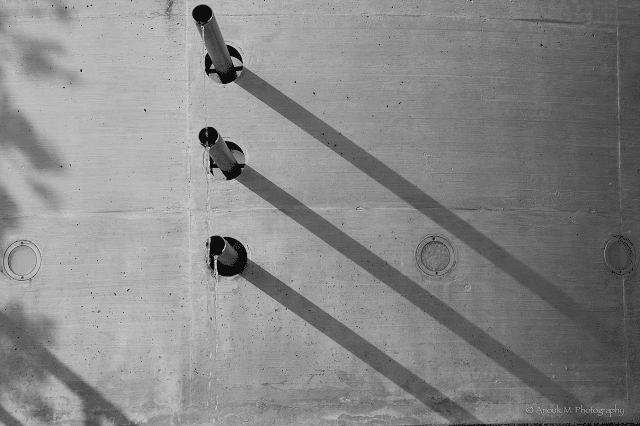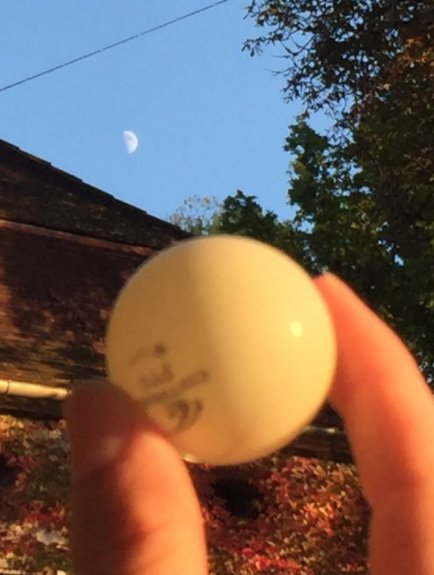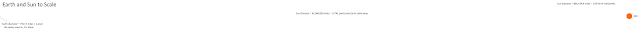This blog is for nonprofit, educational purposes - media is incorporated for educational purposes as outlined in § 107 of the U.S. Copyright Act.

## Wednesday, October 4, 2017

### What do we mean when we say that Sunlight is Parallel?

What we do not mean is that every ray of sunlight is literally and exactly parallel.  This is absurd because the Sun appears as a 1/2° disk in the sky so light from opposite sides of the Sun will form a 1/2° angle here on Earth -- it's that simple.  That is, in fact, why shadows have an umbra (darker inner shadow) and a penumbra.   If you put your eye in the umbra you won't see the Sun, if you put your eye in the penumbral area you'll see some fraction of the Sun, and outside that you'll see the full Sun.

It is exactly the same phenomena as the Umbral area of Totality in a solar eclipse (explored in mathematical detail in my post on calculating the size of the umbra).

If the Sun were closer to the Earth, even 3000 miles away, we would see a pronounced angle between the shadows cast on objects.Figure 1. From Sly Sparkane's excellent YouTube video on Perspective

But we don't see that -- we see extremely parallel shadows.  In fact, they are so parallel that we cannot measure the angle between them.

Remember that the object must be completely vertical and the image taken from directly overhead.  If you angle the object or the camera then the effects of perspective will make it seem like the shadows are angled when they are not.  You have to actually and directly MEASURE the shadows for accuracy, you can't just rely on images that have converted a 3D shape into 2D.  Even this image isn't perfect - but it is only intended to be demonstrative.Figure 2. Shadows are virtually Parallel, even those the edges are angled

And even given the distance between Earth and the Moon, the distance is not sufficient to visually change the shadows cast (the actual difference in the angle of the light here is about 9 arc minutes which is far too small for your eye to notice):Figure 3. Sun angle on Moon vs on Ping Pong ball - very far apart

But the point here is, if the Sun were only "3000" miles away the Sunlight hitting the Moon and that ping pong ball would be ENORMOUSLY different angles.

So what we mean by the "Sunlight is virtually parallel" is that the shadows are parallel.  Even if you place the objects very far apart the "leading edge" of that umbral (or penumbral) shadow will be parallel with the next "leading edge" -- NOT the leading edge of the penumbra with the leading edge of the umbra.

The angle formed between two points some distance apart is actually very easy to calculate because it's a bone simple RISE/RUN calculation.

RISE is the distance between the two points.  Let's say you have two stakes that are 1 meter apart.

RUN is the distance to the Sun in this case.  So that would be 149.6 billion meters.

And to conver the SLOPE of RISE/RUN into an angle you simply use the arctan() function:

arctan(1/149,600,000,000) = 0.0000013788 arcseconds

Measuring even 1 arcsecond is difficult, much less 1/1000000th of an arcsecondFigure 4. Earth and Sun to scale (Earth = 1 pixel)

You can move those stakes 1000 meters apart and you are still looking at 0.00137878 arcseconds -- but now you have accuracy issues in getting your stakes on a perfectly flat surface and perpendicular.  Any error here affects your measurement.

This is why we say the parallax to the Sun is too small to measure and give an accurate distance from the Earth.

To measure the distance to Sun you need other means (see 'Transit of Venus' for example) -- you cannot simply directly measure the angle of Sunlight.

### Conclusion

It is light from the "same part of the Sun" that is virtually parallel over wide areas (angle is too small to measure), NOT that light from opposite edges of the Sun are parallel -- that angle is trivial to measure and is approximately 1/2° (it varies through the year as Earth's distance to the Sun varies due to our elliptical orbit).

This is a clear and direct demonstration of the great distance of the Sun.